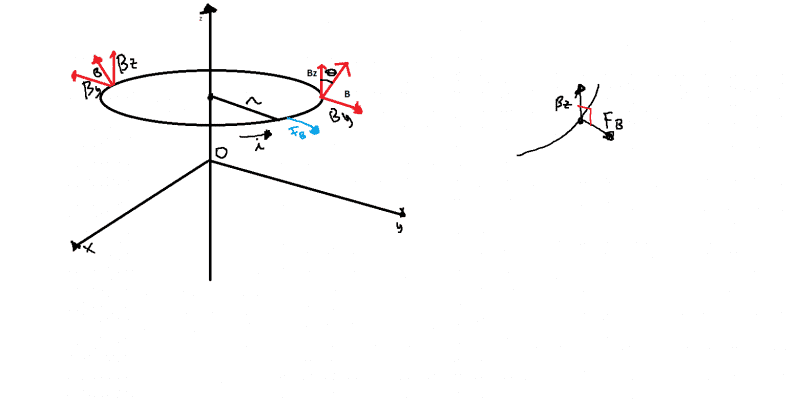# Calculating magnetic force on circular coil carrying current

• cseil

## Homework Statement

A circular coil with center on the z axis and orthogonal to the xy axis carries a current. The coil is in a magnetic field B with axial symmetry compared to the x axis. B forms an angle θ with the z axis. Calculate the magnetic force acting on the coil.2. Homework Equations

$$\vec{F_B} = i \vec{dl} x \vec{B}$$

## The Attempt at a Solution

[/B]
I tried considering $$dl = r d\phi$$
So $F = irB_zd\phi$
where Bz is $Bcos\theta$

By is null because it has opposite verse on opposite points of the coil. So I can consider just Bz

$$F = irBcos\theta d\phi = irBcos\theta \int_0^{2\pi} d\phi = 2\pi irBcos\theta$$

The result, according to the book, is $2\pi irBsin\theta$
Why?

How does your book define ##\theta##? Is it the angle the magnetic field makes with the horizontal(i.e. ##B_y##)?

How does your book define ##\theta##? Is it the angle the magnetic field makes with the horizontal(i.e. ##B_y##)?

It is the angle between B and Bz!

The coil is in a magnetic field B with axial symmetry compared to the x axis.
What does this mean?

What does this mean?

I can't understand what does it mean, actually.

I can't understand what does it mean, actually.
So ask! The problem is not you, it's the instructor who can't write understandably!